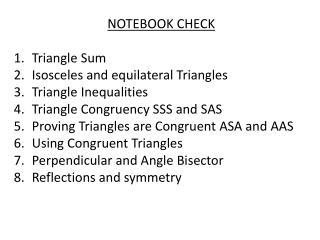DownloadDownload PresentationNOTEBOOK CHECK Triangle Sum Isosceles and equilateral Triangles Triangle Inequalities

# NOTEBOOK CHECK Triangle Sum Isosceles and equilateral Triangles Triangle Inequalities

Télécharger la présentation## NOTEBOOK CHECK Triangle Sum Isosceles and equilateral Triangles Triangle Inequalities

- - - - - - - - - - - - - - - - - - - - - - - - - - - E N D - - - - - - - - - - - - - - - - - - - - - - - - - - -
##### Presentation Transcript

1. NOTEBOOK CHECK Triangle Sum Isosceles and equilateral Triangles Triangle Inequalities Triangle Congruency SSS and SAS Proving Triangles are Congruent ASA and AAS Using Congruent Triangles Perpendicular and Angle Bisector Reflections and symmetry

2. Geometry Unit 3 Review

3. P What is the smallest angle? 14 18 What is the largest angle? R Q 23

4. B In the figure BD bisects AC Name all the congruent parts C A D

5. Find the measure of angle 3? 1 Angle 1 = (2x -3) Angle 2 = (x) Angle 3 = (3x -15) 2 3

6. X Y Given: <U = < Y, XV = VW Prove: UVW = YVW V Make a two Column proof. W U

7. E B < BCA = 47 <EFD = 74 A C D F Line ______ is greater than (>) line ______

8. X Y Given: Line XW and YU bisect each other V Make a two Column proof. W U

9. The exterior angle of a regular polygon is 30. How many sides does the polygon have? What is the measure of the exterior angles of the regular polygon?

10. Determine whether the side lengths can form a triangle • 5, 8, 16 • 20, 24, 40 • 7, 7, 14 • 31, 45, 50

11. X XW // YZ and XY // WZ Prove < W = < Y Y Z W

12. List the sides in order from shortest to longest y 45 108 27 z x

13. What could the length of the missing side of the triangle be 9, 12, ? 10, 15, ? 5, 7, ?

14. What information do you need to prove that the triangles are congruent by SAS? E 75 D C F A 75 B

15. What is the value of X 110 2x + 40 x + 30 65

16. A regular polygon has an interior angle of 135, what would be the sum of the measures of the interior angles.